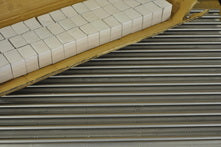As an engineer, I love math. I had a math teacher who said an engineer assumes that a cow is a sphere so he can do calculus integration to determine its volume. There is some truth to that. Engineers, love equations they allow us to do the math easily.

The two common linear rail systems for CNC routers are known as simply supported and fully supported. I could also include fixed support, but the routers I have seen do not give much support.  We will use the simply supported equation to get an idea of the maximum deflection in a router system.

The equation for simply supported beams deflection is Deflection = WL3/48EI where W is the load, L is the Length, E is Young’s Modulus, and I is the inertia. You can find calculators on the internet. I found this one at engineering.com. The round support is the third one down.

If you are looking to design/buy a router with X rail rails are 30" long and the Y axis is 24" long (remember there are 2 rails for the X and 2 rails for the Y). Note the travel distances will be less maybe 24" x 18".

If the rails are 3/4" in diameter and there is 20 lbs of force when as the router plunges into the work, then the maximum X rail deflection is 0.19" and the Y maximum deflection would be 0.09". This means the deflection could be as much as 0.29". This drops to 0.09" if the rail diameters are 1".  If you want to assume that the rails are perfectly fixed at each end you can divide the deflection by 4, but this would be unrealistic for the routers I have seen on the market.

To make matters worse, when the spindle is close to the supports the deflection is less. This means the router will have different errors depending on where it is and how much the tool bit is loaded. This deflection is not just in the Z, but also affects the X and Y axis if we are pushing the bit.